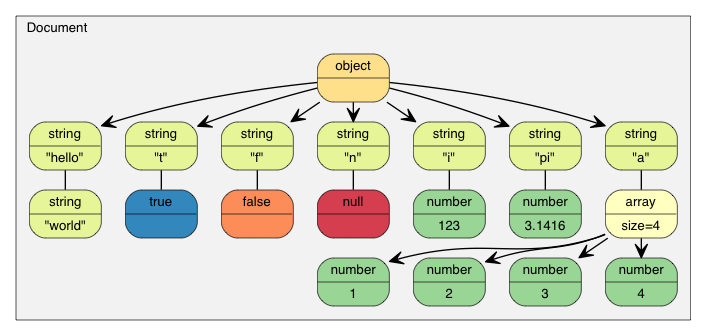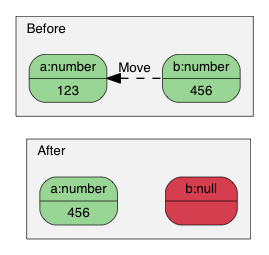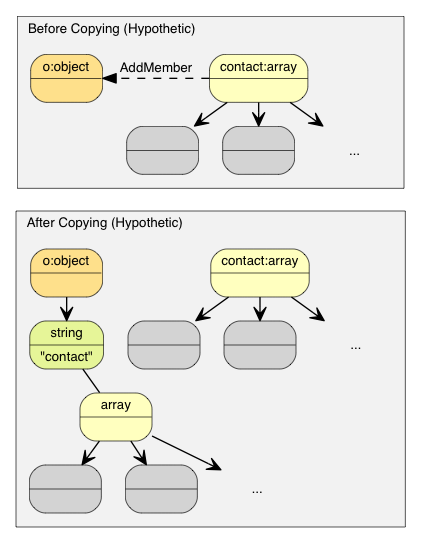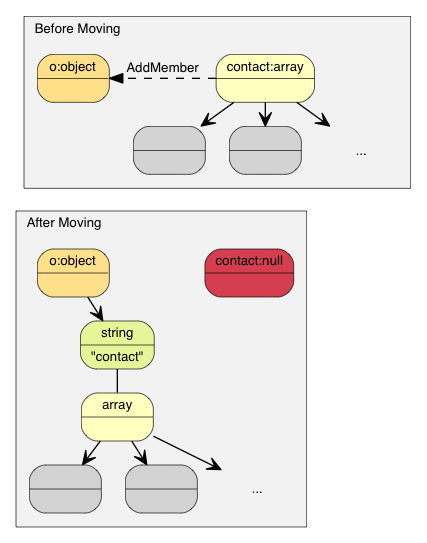# Tutorial

This tutorial introduces the basics of the Document Object Model(DOM) API.

As shown in [Usage at a glance](@ref index), JSON can be parsed into a DOM, and then the DOM can be queried and modified easily, and finally be converted back to JSON.

# Value & Document

Each JSON value is stored in a type called `Value`. A `Document`, representing the DOM, contains the root `Value` of the DOM tree. All public types and functions of RapidJSON are defined in the `rapidjson` namespace.

# Query Value

In this section, we will use excerpt of `example/tutorial/tutorial.cpp`.

Assume we have the following JSON stored in a C string (`const char* json`):

```{
"hello": "world",
"t": true ,
"f": false,
"n": null,
"i": 123,
"pi": 3.1416,
"a": [1, 2, 3, 4]
}
```

Parse it into a `Document`:

```#include "rapidjson/document.h"

using namespace rapidjson;

// ...
Document document;
document.Parse(json);
```

The JSON is now parsed into `document` as a DOM tree:Since the update to RFC 7159, the root of a conforming JSON document can be any JSON value. In earlier RFC 4627, only objects or arrays were allowed as root values. In this case, the root is an object.

```assert(document.IsObject());
```

Let's query whether a `"hello"` member exists in the root object. Since a `Value` can contain different types of value, we may need to verify its type and use suitable API to obtain the value. In this example, `"hello"` member associates with a JSON string.

```assert(document.HasMember("hello"));
assert(document["hello"].IsString());
printf("hello = %s\n", document["hello"].GetString());
```
```hello = world
```

JSON true/false values are represented as `bool`.

```assert(document["t"].IsBool());
printf("t = %s\n", document["t"].GetBool() ? "true" : "false");
```
```t = true
```

JSON null can be queried with `IsNull()`.

```printf("n = %s\n", document["n"].IsNull() ? "null" : "?");
```
```n = null
```

JSON number type represents all numeric values. However, C++ needs more specific type for manipulation.

```assert(document["i"].IsNumber());

// In this case, IsUint()/IsInt64()/IsUInt64() also return true.
assert(document["i"].IsInt());
printf("i = %d\n", document["i"].GetInt());
// Alternative (int)document["i"]

assert(document["pi"].IsNumber());
assert(document["pi"].IsDouble());
printf("pi = %g\n", document["pi"].GetDouble());
```
```i = 123
pi = 3.1416
```

JSON array contains a number of elements.

```// Using a reference for consecutive access is handy and faster.
const Value& a = document["a"];
assert(a.IsArray());
for (SizeType i = 0; i < a.Size(); i++) // Uses SizeType instead of size_t
printf("a[%d] = %d\n", i, a[i].GetInt());
```
```a = 1
a = 2
a = 3
a = 4
```

Note that, RapidJSON does not automatically convert values between JSON types. If a value is a string, it is invalid to call `GetInt()`, for example. In debug mode it will fail an assertion. In release mode, the behavior is undefined.

In the following sections we discuss details about querying individual types.

## Query Array

By default, `SizeType` is typedef of `unsigned`. In most systems, an array is limited to store up to 2^32-1 elements.

You may access the elements in an array by integer literal, for example, `a`, `a`, `a`.

Array is similar to `std::vector`: instead of using indices, you may also use iterator to access all the elements.

```for (Value::ConstValueIterator itr = a.Begin(); itr != a.End(); ++itr)
printf("%d ", itr->GetInt());
```

And other familiar query functions:

• `SizeType Capacity() const`
• `bool Empty() const`

### Range-based For Loop (New in v1.1.0)

When C++11 is enabled, you can use range-based for loop to access all elements in an array.

```for (auto& v : a.GetArray())
printf("%d ", v.GetInt());
```

## Query Object

Similar to Array, we can access all object members by iterator:

```static const char* kTypeNames[] =
{ "Null", "False", "True", "Object", "Array", "String", "Number" };

for (Value::ConstMemberIterator itr = document.MemberBegin();
itr != document.MemberEnd(); ++itr)
{
printf("Type of member %s is %s\n",
itr->name.GetString(), kTypeNames[itr->value.GetType()]);
}
```
```Type of member hello is String
Type of member t is True
Type of member f is False
Type of member n is Null
Type of member i is Number
Type of member pi is Number
Type of member a is Array
```

Note that, when `operator[](const char*)` cannot find the member, it will fail an assertion.

If we are unsure whether a member exists, we need to call `HasMember()` before calling `operator[](const char*)`. However, this incurs two lookup. A better way is to call `FindMember()`, which can check the existence of member and obtain its value at once:

```Value::ConstMemberIterator itr = document.FindMember("hello");
if (itr != document.MemberEnd())
printf("%s\n", itr->value.GetString());
```

### Range-based For Loop (New in v1.1.0)

When C++11 is enabled, you can use range-based for loop to access all members in an object.

```for (auto& m : document.GetObject())
printf("Type of member %s is %s\n",
m.name.GetString(), kTypeNames[m.value.GetType()]);
```

## Querying Number

JSON provides a single numerical type called Number. Number can be an integer or a real number. RFC 4627 says the range of Number is specified by the parser implementation.

As C++ provides several integer and floating point number types, the DOM tries to handle these with the widest possible range and good performance.

When a Number is parsed, it is stored in the DOM as one of the following types:

TypeDescription
`unsigned`32-bit unsigned integer
`int`32-bit signed integer
`uint64_t`64-bit unsigned integer
`int64_t`64-bit signed integer
`double`64-bit double precision floating point

When querying a number, you can check whether the number can be obtained as the target type:

CheckingObtaining
`bool IsNumber()`N/A
`bool IsUint()``unsigned GetUint()`
`bool IsInt()``int GetInt()`
`bool IsUint64()``uint64_t GetUint64()`
`bool IsInt64()``int64_t GetInt64()`
`bool IsDouble()``double GetDouble()`

Note that, an integer value may be obtained in various ways without conversion. For example, A value `x` containing 123 will make `x.IsInt() == x.IsUint() == x.IsInt64() == x.IsUint64() == true`. But a value `y` containing -3000000000 will only make `x.IsInt64() == true`.

When obtaining the numeric values, `GetDouble()` will convert internal integer representation to a `double`. Note that, `int` and `unsigned` can be safely converted to `double`, but `int64_t` and `uint64_t` may lose precision (since mantissa of `double` is only 52-bits).

## Query String

In addition to `GetString()`, the `Value` class also contains `GetStringLength()`. Here explains why.

According to RFC 4627, JSON strings can contain Unicode character `U+0000`, which must be escaped as `"\u0000"`. The problem is that, C/C++ often uses null-terminated string, which treats ``\0'` as the terminator symbol.

To conform RFC 4627, RapidJSON supports string containing `U+0000`. If you need to handle this, you can use `GetStringLength()` to obtain the correct string length.

For example, after parsing a the following JSON to `Document d`:

```{ "s" :  "a\u0000b" }
```

The correct length of the value `"a\u0000b"` is 3. But `strlen()` returns 1.

`GetStringLength()` can also improve performance, as user may often need to call `strlen()` for allocating buffer.

Besides, `std::string` also support a constructor:

```string(const char* s, size_t count);
```

which accepts the length of string as parameter. This constructor supports storing null character within the string, and should also provide better performance.

## Comparing values

You can use `==` and `!=` to compare values. Two values are equal if and only if they are have same type and contents. You can also compare values with primitive types. Here is an example.

```if (document["hello"] == document["n"]) /*...*/;    // Compare values
if (document["hello"] == "world") /*...*/;          // Compare value with literal string
if (document["i"] != 123) /*...*/;                  // Compare with integers
if (document["pi"] != 3.14) /*...*/;                // Compare with double.
```

Array/object compares their elements/members in order. They are equal if and only if their whole subtrees are equal.

Note that, currently if an object contains duplicated named member, comparing equality with any object is always `false`.

# Create/Modify Values

There are several ways to create values. After a DOM tree is created and/or modified, it can be saved as JSON again using `Writer`.

## Change Value Type

When creating a Value or Document by default constructor, its type is Null. To change its type, call `SetXXX()` or assignment operator, for example:

```Document d; // Null
d.SetObject();

Value v;    // Null
v.SetInt(10);
v = 10;     // Shortcut, same as above
```

There are also overloaded constructors for several types:

```Value b(true);    // calls Value(bool)
Value i(-123);    // calls Value(int)
Value u(123u);    // calls Value(unsigned)
Value d(1.5);     // calls Value(double)
```

To create empty object or array, you may use `SetObject()`/`SetArray()` after default constructor, or using the `Value(Type)` in one shot:

```Value o(kObjectType);
Value a(kArrayType);
```

## Move Semantics

A very special decision during design of RapidJSON is that, assignment of value does not copy the source value to destination value. Instead, the value from source is moved to the destination. For example,

```Value a(123);
Value b(456);
b = a;         // a becomes a Null value, b becomes number 123.
```Why? What is the advantage of this semantics?

The simple answer is performance. For fixed size JSON types (Number, True, False, Null), copying them is fast and easy. However, For variable size JSON types (String, Array, Object), copying them will incur a lot of overheads. And these overheads are often unnoticed. Especially when we need to create temporary object, copy it to another variable, and then destruct it.

For example, if normal copy semantics was used:

```Document d;
Value o(kObjectType);
{
Value contacts(kArrayType);
// adding elements to contacts array.
// ...
o.AddMember("contacts", contacts, d.GetAllocator());  // deep clone contacts (may be with lots of allocations)
// destruct contacts.
}
```The object `o` needs to allocate a buffer of same size as contacts, makes a deep clone of it, and then finally contacts is destructed. This will incur a lot of unnecessary allocations/deallocations and memory copying.

There are solutions to prevent actual copying these data, such as reference counting and garbage collection(GC).

To make RapidJSON simple and fast, we chose to use move semantics for assignment. It is similar to `std::auto_ptr` which transfer ownership during assignment. Move is much faster and simpler, it just destructs the original value, `memcpy()` the source to destination, and finally sets the source as Null type.

So, with move semantics, the above example becomes:

```Document d;
Value o(kObjectType);
{
Value contacts(kArrayType);
// adding elements to contacts array.
o.AddMember("contacts", contacts, d.GetAllocator());  // just memcpy() of contacts itself to the value of new member (16 bytes)
// contacts became Null here. Its destruction is trivial.
}
```This is called move assignment operator in C++11. As RapidJSON supports C++03, it adopts move semantics using assignment operator, and all other modifying function like `AddMember()`, `PushBack()`.

### Move semantics and temporary values

Sometimes, it is convenient to construct a Value in place, before passing it to one of the “moving” functions, like `PushBack()` or `AddMember()`. As temporary objects can't be converted to proper Value references, the convenience function `Move()` is available:

```Value a(kArrayType);
Document::AllocatorType& allocator = document.GetAllocator();
// a.PushBack(Value(42), allocator);       // will not compile
a.PushBack(Value().SetInt(42), allocator); // fluent API
a.PushBack(Value(42).Move(), allocator);   // same as above
```

## Create String

RapidJSON provides two strategies for storing string.

1. copy-string: allocates a buffer, and then copy the source data into it.
2. const-string: simply store a pointer of string.

Copy-string is always safe because it owns a copy of the data. Const-string can be used for storing a string literal, and for in-situ parsing which will be mentioned in the DOM section.

To make memory allocation customizable, RapidJSON requires users to pass an instance of allocator, whenever an operation may require allocation. This design is needed to prevent storing a allocator (or Document) pointer per Value.

Therefore, when we assign a copy-string, we call this overloaded `SetString()` with allocator:

```Document document;
Value author;
char buffer;
int len = sprintf(buffer, "%s %s", "Milo", "Yip"); // dynamically created string.
author.SetString(buffer, len, document.GetAllocator());
memset(buffer, 0, sizeof(buffer));
// author.GetString() still contains "Milo Yip" after buffer is destroyed
```

In this example, we get the allocator from a `Document` instance. This is a common idiom when using RapidJSON. But you may use other instances of allocator.

Besides, the above `SetString()` requires length. This can handle null characters within a string. There is another `SetString()` overloaded function without the length parameter. And it assumes the input is null-terminated and calls a `strlen()`-like function to obtain the length.

Finally, for a string literal or string with a safe life-cycle one can use the const-string version of `SetString()`, which lacks an allocator parameter. For string literals (or constant character arrays), simply passing the literal as parameter is safe and efficient:

```Value s;
s.SetString("rapidjson");    // can contain null character, length derived at compile time
s = "rapidjson";             // shortcut, same as above
```

For a character pointer, RapidJSON requires it to be marked as safe before using it without copying. This can be achieved by using the `StringRef` function:

```const char * cstr = getenv("USER");
size_t cstr_len = ...;                 // in case length is available
Value s;
// s.SetString(cstr);                  // will not compile
s.SetString(StringRef(cstr));          // ok, assume safe lifetime, null-terminated
s = StringRef(cstr);                   // shortcut, same as above
s.SetString(StringRef(cstr,cstr_len)); // faster, can contain null character
s = StringRef(cstr,cstr_len);          // shortcut, same as above

```

## Modify Array

Value with array type provides an API similar to `std::vector`.

• `Clear()`
• `Reserve(SizeType, Allocator&)`
• `Value& PushBack(Value&, Allocator&)`
• `template <typename T> GenericValue& PushBack(T, Allocator&)`
• `Value& PopBack()`
• `ValueIterator Erase(ConstValueIterator pos)`
• `ValueIterator Erase(ConstValueIterator first, ConstValueIterator last)`

Note that, `Reserve(...)` and `PushBack(...)` may allocate memory for the array elements, therefore requiring an allocator.

Here is an example of `PushBack()`:

```Value a(kArrayType);
Document::AllocatorType& allocator = document.GetAllocator();

for (int i = 5; i <= 10; i++)
a.PushBack(i, allocator);   // allocator is needed for potential realloc().

// Fluent interface
a.PushBack("Lua", allocator).PushBack("Mio", allocator);
```

This API differs from STL in that `PushBack()`/`PopBack()` return the array reference itself. This is called fluent interface.

If you want to add a non-constant string or a string without sufficient lifetime (see Create String) to the array, you need to create a string Value by using the copy-string API. To avoid the need for an intermediate variable, you can use a temporary value in place:

```// in-place Value parameter
contact.PushBack(Value("copy", document.GetAllocator()).Move(), // copy string
document.GetAllocator());

// explicit parameters
Value val("key", document.GetAllocator()); // copy string
contact.PushBack(val, document.GetAllocator());
```

## Modify Object

The Object class is a collection of key-value pairs (members). Each key must be a string value. To modify an object, either add or remove members. The following API is for adding members:

• `Value& AddMember(Value&, Value&, Allocator& allocator)`
• `Value& AddMember(StringRefType, Value&, Allocator&)`
• `template <typename T> Value& AddMember(StringRefType, T value, Allocator&)`

Here is an example.

```Value contact(kObject);
```

The name parameter with `StringRefType` is similar to the interface of the `SetString` function for string values. These overloads are used to avoid the need for copying the `name` string, since constant key names are very common in JSON objects.

If you need to create a name from a non-constant string or a string without sufficient lifetime (see Create String), you need to create a string Value by using the copy-string API. To avoid the need for an intermediate variable, you can use a temporary value in place:

```// in-place Value parameter
Value().Move(),                                // null value
document.GetAllocator());

// explicit parameters
Value key("key", document.GetAllocator()); // copy string name
Value val(42);                             // some value
```

For removing members, there are several choices:

• `bool RemoveMember(const Ch* name)`: Remove a member by search its name (linear time complexity).
• `bool RemoveMember(const Value& name)`: same as above but `name` is a Value.
• `MemberIterator RemoveMember(MemberIterator)`: Remove a member by iterator (constant time complexity).
• `MemberIterator EraseMember(MemberIterator)`: similar to the above but it preserves order of members (linear time complexity).
• `MemberIterator EraseMember(MemberIterator first, MemberIterator last)`: remove a range of members, preserves order (linear time complexity).

`MemberIterator RemoveMember(MemberIterator)` uses a “move-last” trick to achieve constant time complexity. Basically the member at iterator is destructed, and then the last element is moved to that position. So the order of the remaining members are changed.

## Deep Copy Value

If we really need to copy a DOM tree, we can use two APIs for deep copy: constructor with allocator, and `CopyFrom()`.

```Document d;
Document::AllocatorType& a = d.GetAllocator();
Value v1("foo");
// Value v2(v1); // not allowed

Value v2(v1, a);                      // make a copy
assert(v1.IsString());                // v1 untouched
d.SetArray().PushBack(v1, a).PushBack(v2, a);
assert(v1.IsNull() && v2.IsNull());   // both moved to d

v2.CopyFrom(d, a);                    // copy whole document to v2
assert(d.IsArray() && d.Size() == 2); // d untouched
d.PushBack(v1, a);
```

## Swap Values

`Swap()` is also provided.

```Value a(123);
Value b("Hello");
a.Swap(b);
assert(a.IsString());
assert(b.IsInt());
```

Swapping two DOM trees is fast (constant time), despite the complexity of the trees.

# What's next

This tutorial shows the basics of DOM tree query and manipulation. There are several important concepts in RapidJSON:

1. Streams are channels for reading/writing JSON, which can be a in-memory string, or file stream, etc. User can also create their streams.
2. Encoding defines which character encoding is used in streams and memory. RapidJSON also provide Unicode conversion/validation internally.
3. DOM's basics are already covered in this tutorial. Uncover more advanced features such as in situ parsing, other parsing options and advanced usages.
4. SAX is the foundation of parsing/generating facility in RapidJSON. Learn how to use `Reader`/`Writer` to implement even faster applications. Also try `PrettyWriter` to format the JSON.
5. Performance shows some in-house and third-party benchmarks.
6. Internals describes some internal designs and techniques of RapidJSON.

You may also refer to the FAQ, API documentation, examples and unit tests.